# Two-dimensional hexagonal chromium chalco-halides with large vertical piezoelectricity, high-temperature ferromagnetism, and high magnetic anisotropy†

Wen-Zhi Xiao*a, Liang Xu*b, Gang Xiao a, Ling-Ling Wang c and Xiong-Ying Dai a
aSchool of Science, Hunan Institute of Engineering, Xiangtan 411104, China. E-mail: xiaowenzhi@hnie.edu.cn
bEnergy Materials Computing Center, Jiangxi University of Science and Technology, Nanchang 330013, China. E-mail: liangxu@hnu.edu.cn
cSchool of Physics and Electronics, Hunan University, Changsha 410082, China

Received 29th April 2020 , Accepted 16th June 2020

First published on 17th June 2020

On the basis of density functional theory, we predicted that Janus CrTeI and CrSeBr monolayers possess highly energetic, dynamical, and mechanical stability. Due to noncentral symmetry, the two monolayers exhibit vertical piezoelectricity with large piezoelectric coefficients d31 (1.745 and 1.716 pm V−1 for CrBSe and CrTeI, respectively), which are larger than those of most materials in existence. Both systems are also ferromagnetic (FM) semiconductors, with Curie temperature (TC) higher than 550 K and large in-plane magnetic anisotropy energy. Superexchange interactions are responsible for high-temperature FM order. A semiconductor to half metal transition can be regulated by carrier doping, which can be carried out by gate voltages. Doped systems still retain the same FM order as pristine ones; in particular, hole doping enhances exchange coupling, thereby increasing TC. The combination of piezoelectricity, high TC, and controllable electronic structures and magnetic properties makes magnetic 2D Janus CrSeBr and CrTeI attractive materials for potential applications in nanoelectronics, electromechanics, and spintronics.

## 1. Introduction

Since the discovery of graphene,1 considerable efforts have been made in exploring many other two-dimensional (2D) materials that exhibit novel properties in electronics, spintronics, optics, and magnetism due in part to quantum confinement and dimensionality reduction. The existing 2D materials include graphene,1 BN,2 black phosphorus,3 borophene,4 transition metal dichalcogenides (TMX2),5 and MXenes.6,7 Among them, 2D materials with robust ferromagnetic (FM) order have become a highly desirable target for nanoscale spintronic applications,7–11 but suitable candidates are unavailable because of the lack of intrinsically robust magnetic order or sufficiently high Curie temperature (TC). To date, few 2D magnetic materials have been demonstrated experimentally.12–15 The well-known chromium triiodide (CrI3) monolayer was experimentally found to exhibit Ising ferromagnetism with TC of 45K,12 which is slightly lower than that of its bulk counterpart (61K). Close-to-ideal 2D intrinsic Heisenberg ferromagnetism was observed in the atomic layers of the Cr2Ge2Te6 system in experimental investigation13 and theoretic prediction,16 and the magnetic phase transition temperature was 67.3 K.17–19 The monolayer VSe2 grown on MoS2 exhibits room temperature FM ordering with a large magnetic moment,14,15 which is in accordance with theoretic reports.16,17 FM materials with TC above room temperature are rare in nature, but these discoveries challenge the Mermin–Wagner theorem,20 which predicts that theoretically long-range magnetic order is prohibited in 2D materials at finite temperatures. This phenomenon intrigues scientists into further understanding the underlying physics of 2D magnetic order, and the findings may help in obtaining intrinsic long-range 2D FM materials.

Except for magnetic behavior, piezoelectricity is another particularly interesting and useful property that has attracted tremendous interest.21–26 Piezoelectricity reflects the coupling between mechanical stimulation and electronic output, thereby converting mechanical energy into electricity or vice versa.21,22 Therefore, nanoscale piezoelectric materials are promising candidates for applications in electromechanical sensors, actuators, transducers, and energy harvesters in the fields of nanorobotics, piezotronics, and nanoelectromechanical systems.21–29 From a physical standpoint, piezoelectric polarization can be realized spontaneously in 2D materials by designing materials without central symmetry, indicating that intrinsic piezoelectricity, ferroelasticity, and ferroelectricity exist in such 2D materials. Theoretic advances predict piezoelectric polarizations in 2D Janus transition metal dichalcogenide monolayers (TMXY)23,27 because such materials directly break the inversion and out-of-plane mirror symmetries.27 Experimental observation reported that Janus TMXY, that is, Janus MoSSe, has been successfully realized,28,29 which encourages researchers’ interest in exploring piezoelectric polarizations in Janus TMXY.21–27

Materials with versatile properties offer an avenue for the design of multifunctional nanodevices, thereby extending their applications in various fields. However, at present, there are few reported 2D multifunctional materials that exhibit intrinsic piezoelectricity and ferromagnetism at room temperature. Theoretical calculations predict a kind of 2D Cr-based chalco-halide with lepidocrocite-type structure as FM materials with TC below temperature.30,31 However, out-of-plane piezoelectricity is still lacking because of mirror symmetry. In this study, we designed and investigated hexagonal chromium chalco-halide (CrSeBr and CrTeI) monolayers with Janus MoSSe type structure on the basis of density functional theory (DFT) calculations. The systems under study were magnetic semiconductors, demonstrating large vertical piezoelectricity, high-temperature FM ordering and magnetic anisotropy, and tunable half-metallicity. FM 2D materials in conjunction with their piezoelectricity may result in new physics and innovative device designs for novel applications.

## 2. Computational details

DFT calculations were performed using the Vienna Ab initio Simulation Package,32,33 with projected augmented wave method. We adopted the generalized-gradient exchange–correlation functional within the Perdew–Burke–Ernzerhof (PBE) scheme34 to treat the exchange–correlation energy in all calculations. The energy cutoff for plane wave basis was expanded to 500 eV for the calculation of structural relaxation and electronic structures. The first Brillouin zone (BZ) integration was sampled by 13 × 13 × 1 Monkhorst–Pack k mesh for unit cell. The structures are fully relaxed until the Hellmann–Feynman forces on each atom were less than 0.005 eV Å−1. The convergence criteria for the total energy was set to be 10−6 eV. To eliminate spurious interactions with its periodic images, a vacuum region of approximately 15 Å was applied in the direction perpendicular to the CrXY monolayer. To cope with the correlation effects of the localized Cr-3d electrons, we adopted the GGA + U scheme with the on-site Coulomb U = 3.5 eV and exchange parameters J = 0.7 eV, respectively.35 Phonon dispersion calculations were obtained by the PHONOPY code on the basis of force constant method.36 To ensure the accuracy for magnetic anisotropy energy (MAE) calculation, the BZ of a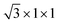rectangular supercell was sampled by Γ-centered Monkhorst–Pack k-point of 10 × 17 × 1, and the convergence criteria for energy increased to 10−8 eV. When evaluating the MAE of monolayers, we considered spin–orbit coupling (SOC).

## 3. Results and discussion

### 3.1 Geometry and stability

The single-layer CrS2 in 1T phase exhibits a hexagonal structure with space group P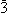m1 (164#),37 whereas CrXY monolayers under study have similar structures with P3m1 space group (156#) due to the absence of out-of-plane mirror symmetry, as shown in Fig. 1(a). Cr atoms are surrounded by six anions to form a distorted octahedral structure. The predicted lattice constants (a) are 3.656 and 3.959 Å at the PBE level for CrSeBr and CrTeI, respectively. The corresponding bond lengths are 2.521, 2.655, 2.755, and 2.861 Å for Cr–Se, Cr–Br, Cr–Te, and Cr–I, respectively. The layer thicknesses (h) are 2.991 and 3.256 Å for CrSeBr and CrTeI, respectively.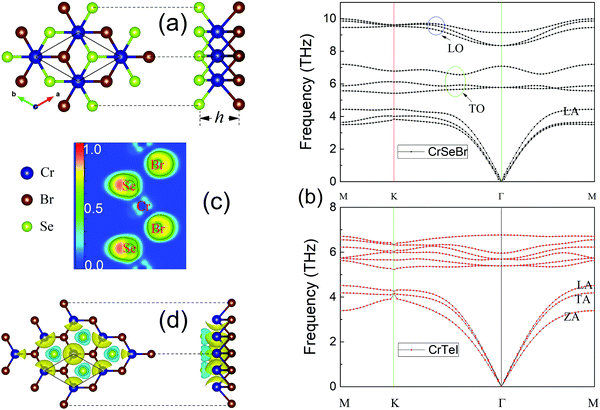Fig. 1 (a) Top and side views of the schematic structures of CrXY monolayer, (b) phonon dispersion spectra of CrSeBr and CrTeI, (c) electron localization function plot of the plane determined by Cr, Se, and Br atoms for CrSeBr monolayer, and (d) spin charge densities of CrSeBr monolayer. The yellow and green correspond to the spin up and spin down, respectively. The isovalue surface level is at 0.01 e Å−3.

To ensure the stability of CrXY, we first considered the binding energy (Eb), which is formulated as Eb = (ECrXYECrEXEY), where ECrXY is the total energy of CrXY and ECr, EX, and EY denote the energy of an isolated atom of Cr, X, and Y in a large enough box, respectively. The calculated Eb at PBE level are −4.721 and −4.01 eV per atom for CrSeBr and CrTeI, respectively. Such large binding energies indicate strong interactions between atoms in CrXY monolayers. To further verify the dynamical stability of the CrXY monolayers, their phonon spectra were investigated, as shown in Fig. 1(b). No negative frequency phonons were observed in the whole BZ for CrXY monolayers. Thus, they should be dynamically stable structures. For CrSeBr, the longitudinal optical (LO) and transverse optical (TO) modes are well separated by a large phonon bandgap of 1.27 THz at the Γ point. A wide phonon bandgap between LO and TO branches indicates a strong ionic nature. This is because the optical mode in ionic crystal induces electric polarization, which in turn increases resilience for the LO branch, thereby resulting in high frequency for the LO branches. Longitudinal acoustic and in- and out-of-plane transverse acoustic (TA and ZA) branches show linear dispersion in the vicinity of the center of the BZ.

To determine the mechanical stability and properties, we investigated the linear elastic constants of all monolayers. For the 2D crystallographic system with hexagonal symmetry, only two independent elastic constants were observed: C11 and C12. C11 is equal to C22, and 2C66 = (C11C12). The corresponding mechanical stability was determined by the Born–Huang criteria:38,39C11 > 0, C66 > 0, and C11 > |C12|. The elastic energy for 2D hexagonal structure can be expressed as follows: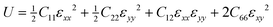.40 The energy-versus-strain plot can be obtained by applying small strains in the range of [−0.02, 0.02] to the unstrained lattice configuration (Fig. S1, ESI). Thus, the elastic constants can be obtained by the polynomial fitting of the strain–energy curves. The calculated elastic constants Cij listed in Table 1 satisfy the mechanical stability criteria for the two monolayers, thereby verifying their mechanical stability. The corresponding Young's moduli are 50.37 and 40.10 N m−1 according to the formula Y = (C112C122)/C11 for CrSeBr and CrTeI, respectively. CrXY systems demonstrate larger mechanical flexibility than those of other well-known 2D materials, such as graphene (340 ± 40 N m−1)41 and MoS2 (126.2 N m−1).42 The significantly low value of Young's moduli in CrXY systems may be ascribed to long and weak bond lengths.

Table 1 Lattice parameters a, elastic coefficients Cij, Young's modulus Y, piezoelectric coefficients eij and dij of CrXY. The units of Cij, eij, and dij for these 2D materials are N m−1, pC m−1, and pm V−1, respectively
Material a (Å) C 11 C 12 Y e 11 e 31 d 11 d 31
CrSeBr 3.656 53.25 12.37 50.37 70.87 115.34 1.733 1.756
CrTeI 3.959 42.74 10.61 40.10 134.52 91.59 4.186 1.716
H-MoS2 3.334 134.3 33.0 126.5 369 3.64
H-MoS242 130 32 126.2 364 3.73
MoSTe49 116 28 109 450 50 5.1 0.4
Te2Se27 38.82 10.49 461.4 12.3 16.285 0.249

### 3.2 Piezoelectricity

Electron localization function (ELF) theory is an effective tool for analyzing chemical bond and characterizing lone pairs.43,44 The ELF value ranges from 0.0 to 1.0. ELF values of 1.00 and 0.50 correspond to perfectly localized and electron-gas-like electrons, respectively, whereas an ELF value of 0.00 refers to extremely low charge density. Taking CrSeBr as an example, we present the ELF in Fig. 1(c). The value around the Cr is approximately 0.25, and the minimum values between the Cr and Se, and Cr and Br are 0.078 and 0.034, respectively, indicating the presence of dominant ionic bonding in this material. An interesting feature is the presence of nonuniform distributions of ELF around the Se and Br sites. The maximum values are 0.88 and 0.78, thereby indicating that mean lone pairs may occur on the outside parts of Br and especially Se planes and suggesting an increased tendency for the emergence of the intrinsic polar electric fields along the normal direction to the monolayer plane due to broken inversion symmetry. This “stereochemical activity of the lone pair” is also a driving force for off-center distortion in magnetoelectric materials.45

Intrinsic polar electric field is a possible reason for the emergence of piezoelectricity. In the CrXY monolayer structure, the difference in atomic size and electronegativity of Te/Se (5.49/5.89, Pearson absolute electronegativity) and I/Br (6.76/7.59) atoms results in inequivalent Cr–Te/Se (2.754/2.521 Å) and Cr–I/Br (2.861/2.655 Å) bonding lengths and charge distributions. The Cr layer is positively charged, whereas I/Br and Te/Se layers are negatively charged. This charge imbalance between Te/Se and I/Br results in a net electric field pointing from the Te/Se layer to the I/Br layer. To identify the inherent electric field further, we plotted the planar charge densities and average of the electrostatic potential (ΔΦ) energy in Fig. 2. We observed changes in ΔΦ, which is associated with the work function change of the structure46,47 and is believed to be proportional to the magnitude of the dipole moment in material, according to the Helmholtz equation.48 The resultant net electric field points from the Te/Se atomic layer to the I/Br atomic layer. The magnitude of the net vertical electric fields are estimated to be 0.197 and 0.229 eV Å−1 for CrSeBr and CrTeI, respectively, following the equation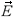= −∇Φ, that is, the slope of the plane-averaged ΔΦ between the two outer most atom minima (shown as the green dashed line in Fig. 2).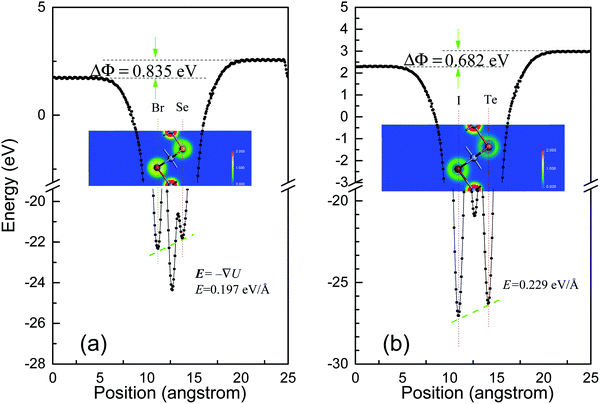Fig. 2 Planar average of the electrostatic potential for (a) CrSeBr and (b) CrTeI monolayers. The insets show the distribution of the charge density in the ground states of CrSeBr and CrTeI. The color indicates the relative amplitude of the local densities.

The lack of inversion symmetry can result in piezoelectric property, which reflects the coupling between electric and mechanical fields in a martial that becomes electrically polarized when subjected to mechanical stress and conversely change shape under an applied electric field. The piezoelectric tensor (eijk) describes the coupling between electrical polarization (Pi) and strain (εjk) tensor. The third-rank tensor is defined as eijk = ∂Pi/∂εjk, where i, j, and k correspond to the x, y, and z Cartesian directions, respectively. eijk consists of two parts, namely, ionic (eionij) and electronic (eelcij) contributions. The piezoelectric strain tensors dij and stress tensor eij relate to each other through the relationship eij = dikCkj, where Ckj is the elastic stiffness tensor. The number of independent components in eij and dij is reduced by the crystal symmetry. For 2D materials with C3v symmetry, eijk has two independent piezoelectric coefficients, namely, e11/d11 and e31/d31. In these equations, e31/d31 describes the polarization induced along the z axis when the crystal is uniformly strained in the basal xy plane, and e11/d11 measures the change in polarization along the x (or y) axis induced by strain in the same direction and accounts for the anisotropy of the electromechanical coupling in the basal xy plane. The dij coefficients of d11 and d31 can be expressed as follows: d11 = e11/(C11C12) and d31 = e31/(C11 + C12).23,26,49

The calculated piezoelectric coefficients eij and dij are listed in Table 1. The 2D coefficient e2Dij must be renormalized by the z lattice parameter that corresponds to the spacing between 2D layers, that is, e2Dij = ze3Dij.26 As a benchmark test, e11 for single-layer MoS2 is 369 pC m−1, which is in excellent agreement with the perilous 364 pC m−1.42 The calculated e11 value of 70.87/134.52 pC m−1 for CrSeBr/CrTeI is much smaller than those reported in 2D TMX226 and Janus TMXY.23,49 However, the obtained e31 values of 115.34 and 91.59 pC m−1 for CrSeBr and CrTeI, respectively, are much larger than those of most TMX2 and Janus TMXY. Given the broken inversion symmetry along the z-direction, the large piezoelectric coefficients of the CrXY monolayer, namely, e31 and d31, are highly desired because they offer freedom to manipulate and design novel piezoelectric devices. The obtained d31 of 1.756/1.716 mp V−1 for CrSeBr/CrTeI is superior to those of most 2D buckled hexagonal III–V compounds,26,50 Janus TMXY,23 Janus group III chalcogenide monolayers, Te2Se (0.249),27 Janus MoSTe (0.4),49 and GaInSe2 (0.46).51 Such a large out-of-plane piezoelectric effect in CrXY makes them potential materials for applications in diverse nanoelectromechanical devices.

### 3.3 Electronic structures and magnetic properties

3.3.1 Electronic structures. To further explore potential applications in the field of spintronics, we illustrate the spin-resolved electronic band structures calculated by the GGA + U in Fig. 3(a) and (b). The CrSeBr and CrTeI systems are semiconductors with the valence band maximum (VBM) at the Γ point and conduction band minimum (CBM) lying between the M and Γ points. The estimated indirect band gaps are 1.414 and 0.494 eV for CrSeBr and CrTeI, respectively. Considering the heavy atomic masses of Te, I, Se, and Br atoms, we calculated the band structure, with the inclusion of the SOC effects, as shown in Fig. 3a′ and b′. The inclusion of the SOC reduces the band gaps to 1.303 and 0.276 eV for CrSeBr and CrTeI, respectively. More interestingly, sizable Zeeman-type valley spin splitting occurs at the Γ point, and the valley spin splitting is 117 and 207 meV for CrSeBr and CrTeI, respectively, at the VBM. The VBM arises mainly from the pxy orbitals of anions, whereas the CBM is composed of the pz state of anions and dxz+yz state of Cr atom. To verify this physical picture, we visualized the real-space wave functions of the CBM and VBM of the monolayers, as shown in Fig. S2 (ESI). The cooperative effect of the out-of-plane magnetic field and SOC of the system itself generates sizable band splitting for pxy at the Γ point. Such large spin splitting provides a possible approach to design a new generation of spintronic devices at room temperature. In addition, we performed calculations within the HSE06 + U method with (and without SOC) to obtain more reliable band gaps. The predicted values are 2.119 (2.246) and 0.875 (1.115) eV for CrSeBr and CrTeI, respectively. The Zeeman-type valley spin splitting changes the values to 127 and 197 meV for CrSeBr and CrTeI, respectively. All the corresponding band structures are available in Fig. S3 and S4 (ESI). In addition to the band gap, we examined the carrier mobilities and optical absorption of the CrXY monolayers using first-principles calculations and deformation potential theory. Fig. S2, S6 and Table SI (ESI) show the calculation results.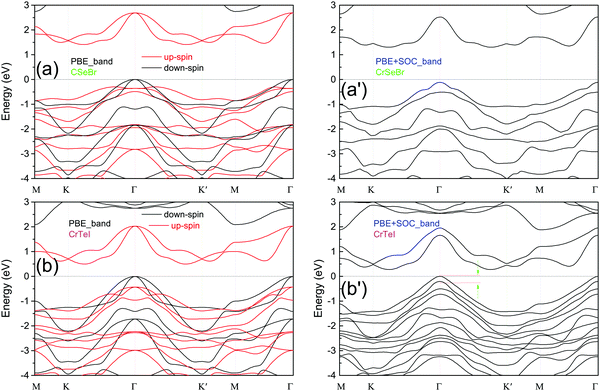Fig. 3 The calculated electronic band structures without and with SOC for (a/a′) CrSeBr monolayer and (b/b′) CrTeI monolayer.
3.3.2 Magnetic moment. The local magnetic moments on Cr sites are 3.510 and 3.729 μB for CrSeBr and CrTeI systems, respectively, as shown in Table 2. The elements in the VIA and VIIA groups are generally negatively bivalent and monovalent, respectively, which render the Cr ion to be in the 3+ state. For 3d ions the crystal field interaction is generally much stronger than the spin–orbit interaction. Therefore, Hund's third rule does not work well. In such distorted octahedral environment, crystal field interaction with ligands results in the quenching of the orbital moment (L = 0). Hence, the orbital moment can be effectively ignored. Thus, the effect magnetic moment for 3d ions can be estimated by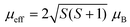rather than by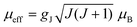. The trivalent Cr has a 3d3 shell with spin angular momentum (S) = 3/2. Hence, the effect magnetic moment is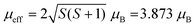, which is consistent with the calculated values above. The local moments on anions are antiparallel to those in Cr sites, and most of them are distributed on the chalcogenide ions, with small contribution from halogen ions, as shown in Table 2 and Fig. 1(c). The antiparallel alignment of the spins between anion and cation sites results in a net magnetic moment of 3.00 μB per CrXY chemical formula and is responsible for FM coupling between Cr sites. The band structure projection of the CrSeBr monolayer further confirms that magnetism mainly originates from the imbalance of the Cr-3d states between the down-spin and up-spin channels, as shown in Fig. 4.
Table 2 Calculated total magnetic moment (Mtotal), and local magnetic moments on Cr (MCr), X (MX) and Y (MY) atoms; exchange parameters of nearest-, and second-neighbor couplings (J1, J2); estimated TC using MC simulations; MAE. The units of magnetic moment, exchange parameters and, MAE are μB, meV and μeV per Cr, respectively
Material M total M Cr M X M Y J 1 J 2 T C (K) MAE
CrSeBr 3.00 3.510 −0.399 −0.075 −6.700 −1.110 550 −186
CrTeI 3.00 3.729 −0.504 −0.117 −8.264 −2.970 956 −1192
CrI3 6.00 3.058 −2.726 47.7 801
CrI354 6.0 3.0 −2.667 804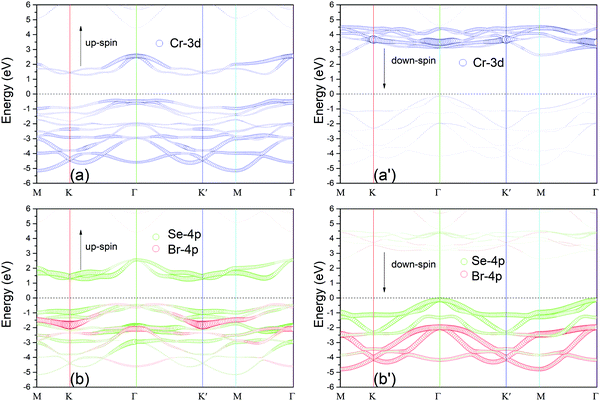Fig. 4 Partial band structure projection of monolayer CrSeBr calculated by the PBE functional without including the SOC effect. The colored symbols show the bands from different atomic orbitals. The size of the symbol is proportional to the weight of the atomic orbitals. The Fermi level is shifted to zero. The arrows ↑ and ↓ corresponds to the up- and down-spin channels, respectively.
3.3.3 T C and magnetic interaction. The magnetic energy gain (ΔEsp) values assessed by the total energy difference between the spin-polarized and non-spin-polarized states are 3.35 eV and 3.49 eV per Cr for CrSeBr and CrTeI, respectively, indicating that the magnetism in both systems are extremely robust. Hence, the exact magnetic ground state should be determined. Four possible collinear magnetic configurations in CrXY monolayers were considered using an orthogonal supercell, as illustrated in Fig. S5 (ESI). The classical spin Heisenberg Hamiltonian is written as follows: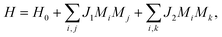where H0 is the nonmagnetic Hamiltonian; Mi is the net magnetic moment at the i site; and J1 and J2 are the nearest-neighbor (NN) and next-nearest-neighbor (NNN) exchange coupling parameters, respectively. According to these magnetic configurations, the NN and NNN exchange coupling parameters J1 and J2 can be obtained, as shown in Table 2. The calculation details are provided in the ESI. The derived J1 and J2 for CrSeBr/CrTeI are −6.750/−8.264 and −1.11/−2.97 meV, respectively. Therefore, the FM interaction between Cr atoms prevails over the anti-ferromagnetic (AFM) interaction, resulting in an energetically preferred FM order in both monolayers. The obtained exchange parameter allows us to further obtain TC by means of mean-field theories or Monte Carlo (MC) simulations with the Metropolis algorithm.52,53 Mean-field theories ignore correlation and fluctuation, thereby overestimating the TC. Hence, relatively reliable MC simulations are adopted. Here, the NN and NNN exchange interactions are only considered for simplification. Although MAE shows a negligible quantity relative to that of exchange coupling, it still is mandatory in physics. For Heisenberg spin Hamiltonian without easy axis, the TC will be predicted to be zero according to the Mermin–Wagner theorem. In the MC simulations, we use 50 × 50 2D lattices that contain 2500 local magnetic moments. The simulations lasted for 5 × 107 loops for each temperature. Fig. 5 presents the relationship of temperature with respect to the average magnetic moment and specific heat (Cv) per chemical formula for CrSeBr and CrTeI monolayers. Through the observation of the variation of the magnetic moment and Cv curves, the magnetic phase transition occurs at approximately 550 and 950 K for CrSeBr and CrTeI monolayers, respectively. This result suggests that the FM state can survive far above the room temperature. To verify the method's validity, we calculated the NN exchange parameter J1 for CrI3 monolayer on the basis of the parameter described in ref. 54. The exchange parameter J1 is 2.726 meV; then, according to triangular lattice, the estimated TC is 47.4 K from MC simulation, which agrees well with the TC value of ∼45 K in the experiment.12 When considered the MAE, the TC was determined to be 35 K and 46 K at the PBE and HSE06 levels, respectivley.55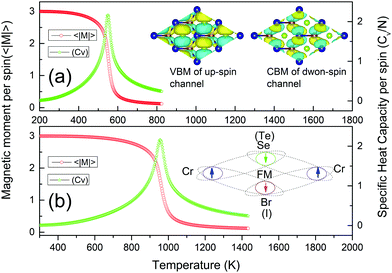Fig. 5 Monte Carlo simulations the average magnetic moment and specific heat Cv with respect to the temperature calculated for the (a) CrSeBr and (b) CrTeI monolayers. Inset shows the wavefunctions at VBM of the down-spin channel (at the upper left) and up-spin channel (at the upper right). The schematic diagram of the exchange mechanism is illustrated at the lower right.

To understand the origin of magnetism, we provide the partial charge density at the VBM for CrSeBr monolayer in Fig. 5. The VBM of the down-spin channel is dominated by Se-4pxy, Br-4pxy, and Cr-3dxz+yz orbitals. The Cr-3dxz+yz orbitals hybridize with the Se-4pxy orbitals, which leads to partial spin pair. Therefore, the AFM coupling through superexchange interaction happens due to the ionicity of the Cr–Se bonds. As a result, the ⋯–Cr↑–Se↓–Cr↑–⋯ coupling chain induces robust FM order, as shown in the lower right panel of Fig. 5. For the up-spin channel, the Cr-3dxz+yz orbitals hybridize with the Br-4pxy ones at the VBM. Thus, ⋯–Cr↑–Br↓–Cr↑–⋯ coupling chain works in the same manner.

3.3.4 MAE. MAE inhibits magnetization reversal in materials; hence, it is important for stabilizing long-range magnetic order56 and closely related to information storage. To evaluate the magnetic anisotropy, we consider the SOC effect when the noncollinear magnetic calculations are carried out. To search the easy axis, which is an energetically preferred axis for the spontaneous magnetization, we first performed a series of self-consistent calculations with magnetization direction parallel or perpendicular to the monolayers. MAE is generally defined as MAE = EE, where E and E correspond to the total energies of the noncollinear non-self-consistent calculations with magnetization directions parallel and perpendicular to the plane of monolayer, respectively. According to a rectangular cell of the 2D CrXY monolayer, as shown in Fig. 6, the angular-dependent MAE can be expressed as follows:57 MAE = K1sin2θ + K2sin4θ, where K1 and K2 are the anisotropy constants and θ is the azimuthal angle of rotation. Anisotropy constants will be obtained by fitting the MAE–θ curve to this equation. As illustrated in Fig. 6, the energy is isotropic in the xy plane, whereas the MAE strongly is sensitive to the magnetization direction in either the yz or xz plane. Therefore, both systems are of magnetic anisotropy with in-plane easy magnetization axis.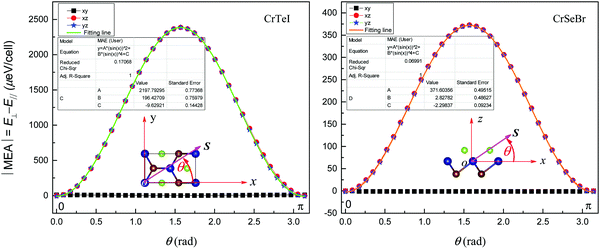Fig. 6 Dependence of the absolute value of MAE on the direction of magnetization for (a) CrTeI and (b) CrSeBr monolayers. The inset illustrates that the spin vector S on the xy, and xz plane is rotated with an angle θ about the x axis. The rectangular cell used for calculations contains two Cr atoms.

As shown in Table 2, the MAE values are −186 and −1192 μeV per Cr atom for CrSeBr and CrTeI systems, respectively. Large MAE in CrTeI results from strong SOC because Te and I atoms are heavier than Se and Br atoms. Such MAE is larger than the reported values of other intrinsic 2D FM materials, such as VOCl2 (16.6 μeV per V),58 CrI3 (803.65 μeV per Cr),54 and CrGeTe3 (20 μeV per Cr).59 For a system containing a heavy element, the MAE stems from the cooperative effect of the anisotropy in the atom's orbital moment L and its interaction with the spin angular momentum S.60–62 For Cr-based FM materials, Lado et al.63 suggested that large MAE in CrI3 stems from the strong SOC of the heavy iodine ions, whereas Zhang et al.64 attributed the MAE to the interaction of I-px/py and Cr-dxy/dx2y2 orbitals to Cr2I3X3 (X = Br, Cl) monolayers.

According to the perturbation theory, Wang et al.65 interpreted MAE as the competition between vertical and in-plane SOC effects. Hence, MAE is defined as follows: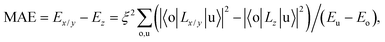where ξ is the c strength of SOC; o and u denote the occupied and unoccupied states of the same spin, that is, up–up (↑↑) or down–down (↓↓), respectively; Eo and Eu are the energy levels of the occupied and unoccupied states, respectively; and Lz and Lx/y are the angular momentum operators along the z and x/y directions, respectively. To understand the origin of the large magnetic anisotropy, we demonstrate the projected density of states of the Cr-3d and p orbitals of the anion in Fig. 7. For both systems, the interactions between the pz and dz2 and between the dxz and dyz orbitals contribute to the positive MAE in the up-spin channel. The positive contribution from the down-spin channel is marginal due to the large value of EuEo and small DOS near the Fermi level, especially for the 3d orbitals. Negative MAE is attributed to SOC interaction among the occupied px/y, dxz, dyz, and dx2+y2 orbitals in the down-spin channel with those unoccupied orbitals in the up-spin channel. EuEo is much smaller than EuEo, thereby indicating that negative MAE dominates over positive MAE.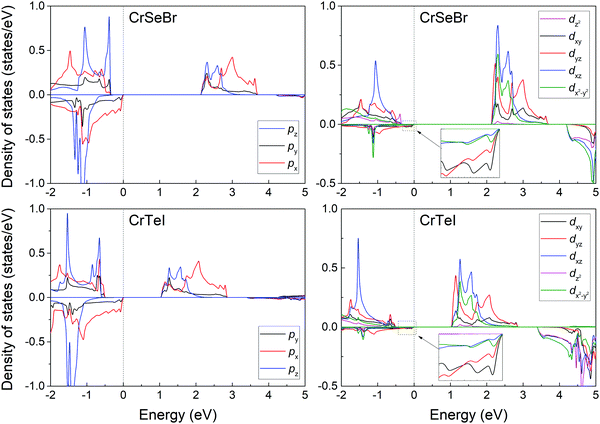Fig. 7 The projected DOS of Cr-3d orbitals and p orbitals of anions for (a) CrTeI and (b) CrSeBr monolayers.
3.3.4 Controlling electronic structures and magnetism by carrier doping. VBs in the down-spin channel and CBs in the up-spin channel simultaneously approach the Fermi level. Therefore, the CrXY monolayers are typical bipolar magnetic semiconductor materials.66,67 The unique electronic structures provide promising ways to realize half-metallicity and manipulate fully spin-polarized currents in a specific spin channel by adjusting the position of the Fermi level. To reach such aim, we dope holes or electrons into these two systems with a realizable doping level at 0.1|e| per unit cell.11 As expected, under hole doping, both systems change form semiconductors into half metals with completely spin polarization in down-spin channel (Fig. 8(a) and (b)). At the same time, the hole doping results in the same transition with full polarization in the up-spin channel (Fig. 8(a′) and (b′)). In practice, electrical gating technique can be applied on the CrXY monolayers to tailor the position of the Fermi level.11,66,67Fig. 8(c) shows the schematic of the bipolar field-effect spin-filter device on the basis of the CrXY systems. When a positive gate voltage (VG > 0) is applied, the Fermi level shifts into the VB, and the fully polarized currents will appear in the down-spin channel.67 Upon the application of a negative gate voltage (VG < 0), the Fermi level moves up into the CB, and completely polarized currents will appear in the up-spin channel. Therefore, completely spin-polarized currents with tunable spin polarization can be realized by applying a voltage gate upon the CrXY systems.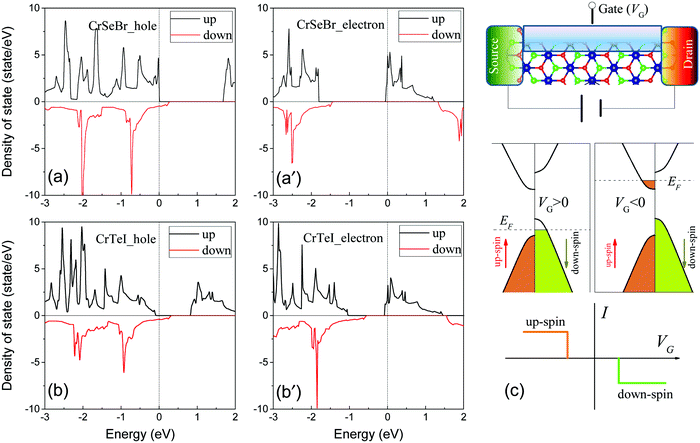Fig. 8 The total density of states for the (a) CrSeBr and (b) CrTeI under hole doping at a doping concentration of 0.1 carrier per unit cell. The right panels show the DOSs under electron doping at the same concentration as hole for (a′) CrSeBr and (b′) CrTeI. (c) Schematic of the bipolar field-effect spin-filter device and its I–VG relationship. The Fermi levels are set to zero.

Carrier doping can modify the electronic structures and induce magnetic phase transition for a magnetic system.11,66 Thus, whether the doped systems still retain room temperature FM ordering is an extremely interesting research topic. We provide the exchange parameters J1 and J2 in Table 3 with carrier doping concentrations varying from −0.2 to 0.2|e| per unit cell. Electron and hole doping result in equal numbers of increment and decrement of magnetic moments, respectively. Thus, magnetization is minimally affected because there is a total magnetic moment of 3.0 μB per unit cell. According to the variation of the exchange parameters J1 and J2, FM interaction monotonically increases when the doping level changes from −0.2|e| to 0.2|e| per unit cell. Hole doping is favorable toward enhancing FM interaction, thereby promoting TC. Meanwhile, electron doping plays the opposite role. Although the concentration of electron doping increases up to 0.1 electrons per unit cell, the estimated TC (378 K) is well above room temperature for CrSeBr. The result reveals a feasible way to realize room temperature half-metallicity by a suitable gate voltage.

Table 3 Calculated first and second nearest exchange couplings parameters J1 and J2 for CrSeBr and CrTeI monolayers doped with carriers at different doping level. The unit is meV for exchange couplings parameters
Structure Carriers doping concentration (|e| per unit cell)
−0.2 −0.1 0.0 0.1 0.2
J 1 J 2 J 1 J 2 J 1 J 2 J 1 J 2 J 1 J 2
CrSeBr −3.19 1.29 −4.77 0.33 −6.70 −1.11 −7.49 −2.36 −8.21 −3.63
CrTeI −4.60 −0.27 −6.16 −1.04 −8.26 −2.97 −8.49 −3.18 −8.22 −3.34

## 4. Conclusions

We have demonstrated stability, strong piezoelectric effects, high temperature ferromagnetism with robust magnetic anisotropy, and tunable electronic structures in Janus CrSeBr and CrTeI monolayers with triangular lattice through first-principles simulations. Given the lack of reflection symmetry, both Janus monolayers show vertical piezoelectric polarizations characterized by d31, which are comparable to or even superior to most 2D piezoelectric materials, such as Janus TMXY. The two magnetic semiconducting materials also possess TC that is much higher than room temperature on the basis of MC simulations and exhibit considerably large in-plane MAE values of 186 and 1192 μeV per Cr atom. Carrier doping, regardless of the carrier type, induces transition from magnetic semiconductor to half metal. Particularly, hole doping improves FM interaction, thereby promoting the TC of the systems. These results enrich the diversity of the Janus 2D materials, which have potential applications in electromechanical and spintronic devices in nanoscale.

## Conflicts of interest

There are no conflicts to declare.

## Acknowledgements

This work was supported by the National Natural Science Foundation of China (No. 51701071), and the Scientific Research Fund of Hunan Provincial Education Department (No. 18A347 and 19C0487). We acknowledge the computational support provided by the computing platform of the Network Information Center of Hunan Institute of Engineering.

## Notes and references

1. K. S. Novoselov, A. K. Geim, S. V. Morozov, D. Jiang, Y. Zhang, S. V. Dubonos, I. V. Grigorieva and A. A. Firsov, Electric field effect in atomically thin carbon films, Science, 2004, 306(5696), 666–669 CrossRef CAS PubMed.
2. K. S. Novoselov, D. Jiang, F. Schedin, T. Booth, V. V. Khotkevich, S. V. Morozov and A. K. Geim, Two-dimensional atomic crystals, Proc. Natl. Acad. Sci. U. S. A., 2005, 102(30), 10451–10453 CrossRef CAS PubMed.
3. W. Lu, H. Nan, J. Hong, Y. Chen, C. Zhu, Z. Liang, X. Ma, Z. Ni, C. Jin and Z. Zhang, Plasma-assisted fabrication of monolayer phosphorene and its Raman characterization, Nano Res., 2014, 7(6), 853–859 CrossRef CAS.
4. W. Li, L. Kong, C. Chen, J. Gou, S. Sheng, W. Zhang, H. Li, L. Chen, P. Cheng and K. Wu, Experimental realization of honeycomb borophene, Sci. Bull., 2018, 63(5), 282–286 CrossRef CAS.
5. X. Qian, J. Liu, L. Fu and J. Li, Quantum spin Hall effect in two-dimensional transition metal dichalcogenides, Science, 2014, 346(6215), 1344–1347 CrossRef CAS PubMed.
6. M. Naguib, V. Mochalin, M. W. Barsoum and Y. Gogotsi, 25th anniversary article: MXenes: a new family of two-dimensional materials, Adv. Mater., 2014, 26(7), 992–1005 CrossRef CAS PubMed.
7. J. Yang, S. Zhang, A. Wang, R. Wang, C. Wang, G. Zhang and L. Chen, High magnetoresistance in ultra-thin two-dimensional Cr-based MXenes, Nanoscale, 2018, 10(41), 19492–19497 RSC.
8. L. Dong, H. Kumar, B. Anasori, Y. Gogotsi and V. B. Shenoy, Rational design of two-dimensional metallic and semiconducting spintronic materials based on ordered double-transition-metal MXenes, J. Phys. Chem. Lett., 2017, 8(2), 422–428 CrossRef CAS PubMed.
9. A. Soumyanarayanan, N. Reyren and A. Fert, et al., Emergent phenomena induced by spin–orbit coupling at surfaces and interfaces, Nature, 2016, 539(7630), 509–517 CrossRef CAS PubMed.
10. W. Zhang, Q. Qu, P. Zhu and C. Lam, Robust intrinsic ferromagnetism and half semiconductivity in stable two-dimensional single-layer chromium trihalides, J. Mater. Chem. C, 2015, 3(48), 12457–12468 RSC.
11. S. Jiang, L. Li, Z. Wang, K. F. Mak and J. Shan, Controlling magnetism in 2D CrI3 by electrostatic doping, Nat. Nanotechnol., 2018, 13(7), 549–553 CrossRef CAS PubMed.
12. B. Huang, G. Clark, E. Navarromoratalla, D. R. Klein, R. Cheng, K. L. Seyler, D. Zhong, E. R. Schmidgall, M. A. Mcguire, D. Cobden, W. Yao, D. Xiao, P. Jarilloherrero and X. Xu, Layer-dependent ferromagnetism in a van der Waals crystal down to the monolayer limit, Nature, 2017, 546(7657), 270–273 CrossRef CAS PubMed.
13. C. Gong, L. Li, Z. Li, H. Ji, A. Stern, Y. Xia, T. Cao, W. Bao, C. Wang, Y. Wang, Z. Q. Qiu, R. J. Cava, S. G. Louie, J. Xia and X. Zhang, Discovery of intrinsic ferromagnetism in two-dimensional van der Waals crystals, Nature, 2017, 546(7657), 265–269 CrossRef CAS PubMed.
14. M. Bonilla, S. Kolekar, Y. Ma, H. C. Diaz, V. Kalappattil, R. Das, T. Eggers, H. R. Gutierrez, M.-H. Phan and M. Batzill, Strong room-temperature ferromagnetism in VSe2 monolayers on van der Waals substrates, Nat. Nanotechnol., 2018, 13(4), 289–293 CrossRef CAS PubMed.
15. Z.-L. Liu, X. Wu, Y. Shao, J. Qi, Y. Cao, L. Huang, C. Liu, J.-O. Wang, Q. Zheng, Z.-L. Zhu, K. Ibrahim, Y.-L. Wang and H.-J. Gao, Epitaxially grown monolayer VSe2: an air-stable magnetic two-dimensional material with low work function at edges, Sci. Bull., 2018, 63, 419–425 CrossRef CAS.
16. Y. Ma, Y. Dai, M. Guo, C. Niu, Y. Zhu and B. Huang, Evidence of the existence of magnetism in pristine VX2 monolayers (X = S, Se) and their strain-induced tunable magnetic properties, ACS Nano, 2012, 6(2), 1695–1701 CrossRef CAS PubMed.
17. H.-R. Fuh, C.-R. Chang, Y.-K. Wang, R. F. L. Evans, Roy W. Chantrell and H.-T. Jeng, Newtype single-layer magnetic semiconductor in transition-metal dichalcogenides VX2 (X = S, Se and Te), Sci. Rep., 2016, 6, 32625 CrossRef CAS PubMed.
18. Z. Hao, H. Li, S. Zhang, X. S. Li, G. Lin, X. Luo, Y. Sun, Z. Liu and Y. Wang, Atomic scale electronic structure of the ferromagnetic semiconductor Cr2Ge2Te6, Sci. Bull., 2018, 63(13), 825–830 CrossRef CAS.
19. G. T. Lin, H. L. Zhuang, X. Luo, B. J. Liu, F. C. Chen, J. Yan, Y. Sun, J. Zhou, W. J. Lu, P. Tong, Z. G. Sheng, Z. Qu, W. H. Song, X. B. Zhu and Y. P. Sun, Tricritical behavior of two-dimensional intrinsic ferromagnetic semiconducting CrGeTe3, arXiv preprint 2017, arXiv:1706.03239.
20. N. D. Mermin and H. Wagner, Absence of ferromagnetism or antiferromagnetism in one- or two-dimensional isotropic Heisenberg models, Phys. Rev. Lett., 2016, 17, 1133–1136 CrossRef.
21. P. Lin, C. Pan and Z. L. Wang, Two-dimensional nanomaterials for novel piezotronics and piezophototronics, Mater. Today Nano, 2018, 4, 17–31 CrossRef.
22. S. Kang, S. Kim, S. Jeon, W. Jang, D. Seol, Y. Kim, J. Lee, H. Yang and Y. Kim, Atomic-scale symmetry breaking for out-of-plane piezoelectricity in two-dimensional transition metal dichalcogenides, Nano Energy, 2019, 58, 57–62 CrossRef CAS.
23. L. Dong, J. Lou and V. B. Shenoy, Large in-plane and vertical piezoelectricity in Janus transition metal dichalchogenides, ACS Nano, 2017, 11(8), 8242–8248 CrossRef CAS PubMed.
24. J. Yang, A. Wang, S. Zhang, J. Liu, Z. Zhong and L. Chen, Coexistence of piezoelectricity and magnetism in two-dimensional vanadium dichalcogenides, Phys. Chem. Chem. Phys., 2019, 21(1), 132–136 RSC.
25. C. Zhang, Y. Nie, S. Sanvito and A. Du, First-principles prediction of a room-temperature ferromagnetic Janus VSSe monolayer with piezoelectricity, ferroelasticity, and large valley polarization, Nano Lett., 2019, 19(2), 1366–1370 CrossRef PubMed.
26. M. N. Blonsky, H. L. Zhuang, A. K. Singh and R. G. Hennig, Ab initio prediction of piezoelectricity in two-dimensional materials, ACS Nano, 2015, 9(10), 9885–9891 CrossRef CAS PubMed.
27. Y. Chen, J. Liu, J. Yu, Y. Guo and Q. Sun, Symmetry-breaking induced large piezoelectricity in Janus tellurene materials, Phys. Chem. Chem. Phys., 2019, 21(3), 1207–1216 RSC.
28. A.-Y. Lu, H. Zhu, J. Xiao, C.-P. Chuu, Y. Han, M.-H. Chiu, C.-C. Cheng, C.-W. Yang, K.-H. Wei, Y. Yang, Y. Wang, D. Sokaras, D. Nordlund, P. Yang, D. A. Muller, M.-Y. Chou, X. Zhang and L.-J. Li, Janus monolayers of transition metal dichalcogenides, Nat. Nanotechnol., 2017, 12(8), 744–749 CrossRef CAS PubMed.
29. J. Zhang, S. Jia, I. Kholmanov, L. Dong, D. Er, W. Chen, H. Guo, Z. Jin, V. B. Shenoy, L. Shi and J. Lou, Janus monolayer transition-metal dichalcogenides, ACS Nano, 2017, 11(8), 8192–8198 CrossRef CAS PubMed.
30. C. Wang, X. Zhou, L. Zhou, N. Tong, Z. Lu and W. Ji, A family of high-temperature ferromagnetic monolayers with locked spin-dichroism-mobility anisotropy: MnNX and CrCX (X = Cl, Br, I; C = S, Se, Te), Sci. Bull., 2019, 64(5), 293–300 CrossRef CAS.
31. N. Miao, B. Xu, L. Zhu, J. Zhou and Z. Sun, 2D intrinsic ferromagnets from van der Waals antiferromagnets, J. Am. Chem. Soc., 2018, 140, 2417–2420 CrossRef CAS PubMed.
32. G. Kresse and J. Furthmüller, Efficient iterative schemes for ab initio total-energy calculations using a plane-wave basis set, Phys. Rev. B: Condens. Matter Mater. Phys., 1996, 54(16), 11169 CrossRef CAS PubMed.
33. D. Hobbs, G. Kresse and J. Hafner, Fully unconstrained noncollinear magnetism within the projector augmented-wave method, Phys. Rev. B: Condens. Matter Mater. Phys., 2000, 62(17), 11556 CrossRef CAS.
34. J. P. Perdew, K. Burke and M. Ernzerhof, Generalized gradient approximation made simple, Phys. Rev. Lett., 1996, 77(18), 3865 CrossRef CAS PubMed.
35. M. Cococcioni and S. De Gironcoli, Linear response approach to the calculation of the effective interaction parameters in the LDA + U method, Phys. Rev. B: Condens. Matter Mater. Phys., 2005, 71(3), 035105 CrossRef.
36. A. Togo, F. Oba and I. Tanaka, First-principles calculations of the ferroelastic transition between rutile-type and CaCl2-type SiO2 at high pressures, Phys. Rev. B: Condens. Matter Mater. Phys., 2008, 78(13), 134106 CrossRef.
37. H. L. Zhuang, M. D. Johannes, M. N. Blonsky and R. G. Hennig, Computational prediction and characterization of single-layer CrS2, Appl. Phys. Lett., 2014, 104(2), 022116 CrossRef.
38. M. Born and K. Huang, Dynamical Theory of Crystal Lattices Oxford Classic Texts in the Physical Sciences, Clarendon, Oxford, 1988 Search PubMed.
39. Z. Wu, E. Zhao, H. Xiang, X. Hao, X. Liu and J. Meng, Crystal structures and elastic properties of superhard IrN2 and IrN3 from first principles, Phys. Rev. B: Condens. Matter Mater. Phys., 2007, 76(5), 054115 CrossRef.
40. E. Cadelano, P. L. Palla, S. Giordano and L. Colombo, Elastic properties of hydrogenated graphene, Phys. Rev. B: Condens. Matter Mater. Phys., 2010, 82(23), 235414 CrossRef.
41. C. Lee, X. g Wei, J. W. Kysar and J. Hone, Measurement of the elastic properties and intrinsic strength of monolayer graphene, Science, 2008, 321(5887), 385–388 CrossRef CAS PubMed.
42. K. A. N. Duerloo, M. T. Ong and E. J. Reed, Intrinsic piezoelectricity in two-dimensional materials, J. Phys. Chem. Lett., 2012, 3(19), 2871–2876 CrossRef CAS.
43. A. D. Becke and K. E. Edgecombe, A simple measure of electron localization in atomic and molecular systems, J. Chem. Phys., 1990, 92(9), 5397–5403 CrossRef CAS.
44. D. B. Chesnut, An electron localization function study of the lone pair, J. Phys. Chem. A, 2000, 104(49), 11644–11650 CrossRef CAS.
45. P. Ravindran, R. Vidya, A. Kjekshus, H. Fjellvag and O. Eriksson, Theoretical investigation of magnetoelectric behavior in BiFeO3, Phys. Rev. B: Condens. Matter Mater. Phys., 2006, 74(22), 224412 CrossRef.
46. Y. Ma, Y. Dai, W. Wei, X. Li and B. Huang, Emergence of electric polarity in BiTeX (X = Br and I) monolayers and the giant Rashba spin splitting, Phys. Chem. Chem. Phys., 2014, 16(33), 17603–17609 RSC.
47. Q. Yao, J. Cai, W. Tong, S. Gong, J. Wang, X. Wan, C. Duan and J. Chu, Manipulation of the large Rashba spin splitting in polar two-dimensional transition-metal dichalcogenides, Phys. Rev. B: Condens. Matter Mater. Phys., 2017, 95(16), 165401 CrossRef.
48. R. Dautray and J. L. Lions, Mathematical Analysis and Numerical Methods for Science and Technology, Volume 4: Integral Equations and Numerical Methods, Springer, Berlin, 2000 Search PubMed.
49. M. Yagmurcukardes, C. Sevik and F. M. Peeters, Electronic, vibrational, elastic, and piezoelectric properties of monolayer Janus MoSTe phases: A first-principles study, Phys. Rev. B: Condens. Matter Mater. Phys., 2019, 100(4), 045415 CrossRef CAS.
50. R. Gao and Y. Gao, Piezoelectricity in two-dimensional group III-V buckled honeycomb monolayers, Phys. Status Solidi RRL, 2017, 11(3), 1600412 CrossRef.
51. Y. Guo, S. Zhou, Y. Bai and J. Zhao, Enhanced piezoelectric effect in Janus group-III chalcogenide monolayers, Appl. Phys. Lett., 2017, 110(16), 163102 CrossRef.
52. N. Metropolis, A. W. Rosenbluth, M. N. Rosenbluth, A. H. Teller and E. Teller, Equation of state calculations by fast computing machines, J. Chem. Phys., 1953, 21(6), 1087–1092 CrossRef CAS.
53. J. Kotze, Introduction to Monte Carlo methods for an Ising Model of a Ferromagnet, arXiv preprint 2008, arXiv:0803.0217.
54. L. Webster and J. A. Yan, Strain-tunable magnetic anisotropy in monolayer CrCl3, CrBr3, and CrI3, Phys. Rev. B: Condens. Matter Mater. Phys., 2018, 98(14), 144411 CrossRef CAS.
55. Y. Zhao, L. Lin, Q. Zhou, Y. Li, S. Yuan, Q. Chen, S. Dong and J. Wang, Surface Vacancy-Induced Switchable Electric Polarization and Enhanced Ferromagnetism in Monolayer Metal Trihalides, Nano Lett., 2018, 18, 2943–2949 CrossRef CAS PubMed.
56. P. Gambardella, S. Rusponi, M. Veronese, S. S. Dhesi, C. Grazioli, A. Dallmeyer, I. Cabria, R. Zeller, P. H. Dederichs, K. Kern, C. Carbone and H. Brune, Giant magnetic anisotropy of single cobalt atoms and nanoparticles, Science, 2003, 300(5622), 1130–1133 CrossRef CAS PubMed.
57. A. N. Bogdanov and I. E. Dragunov, Metastable states, spin–reorientation transitions, and domain structures in planar hexagonal antiferromagnets, Low Temp. Phys., 1998, 24(12), 852–857 CrossRef CAS.
58. H. Ai, X. Song, S. Qi, W. Li and M. Zhao, Intrinsic multiferroicity in two-dimensional VOCl2 monolayers, Nanoscale, 2019, 11(3), 1103–1110 RSC.
59. C. Xu, J. Feng, H. Xiang and L. Bellaiche, Interplay between Kitaev interaction and single ion anisotropy in ferromagnetic CrI3 and CrGeTe3 monolayers, npj Comput. Mater., 2018, 4(1), 1–6 CrossRef CAS.
60. Y. Zhang, Z. Wang and J. Cao, Prediction of magnetic anisotropy of 5d transition metal-doped gC3N4, J. Mater. Chem. C, 2014, 2(41), 8817–8821 RSC.
61. P. Wang, X. Jiang, J. Hu, X. Huang and J. Zhao, Giant magnetic anisotropy of a 5d transition metal decorated two-dimensional polyphthalocyanine framework, J. Mater. Chem. C, 2016, 4(11), 2147–2154 RSC.
62. J. Zhou, Q. Wang, Q. Sun, Y. Kawazoe and P. Jena, Giant magnetocrystalline anisotropy of 5d transition metal-based phthalocyanine sheet, Phys. Chem. Chem. Phys., 2015, 17(26), 17182–17189 RSC.
63. J. L. Lado and J. Fernández-Rossier, On the origin of magnetic anisotropy in two dimensional CrI3, 2D Mater., 2017, 4(3), 035002 CrossRef.
64. F. Zhang, W. Mi and X. Wang, Spin-Dependent Electronic Structure and Magnetic Anisotropy of 2D Ferromagnetic Janus Cr2I3X3 (X = Br, Cl) Monolayers, Adv. Electron. Mater., 2020, 6(1), 1900778 CrossRef CAS.
65. D. Wang, R. Wu and A. J. Freeman, First-principles theory of surface magnetocrystalline anisotropy and the diatomic-pair model, Phys. Rev. B: Condens. Matter Mater. Phys., 1993, 47(22), 14932 CrossRef CAS PubMed.
66. X. Li, X. Wu, Z. Li, J. Yang and J. G. Hou, Bipolar magnetic semiconductors: a new class of spintronics materials, Nanoscale, 2012, 4(18), 5680–5685 RSC.
67. X. Li and J. Yang, Bipolar magnetic materials for electrical manipulation of spin-polarization orientation, Phys. Chem. Chem. Phys., 2013, 15(38), 15793–15801 RSC.

### Footnote

Electronic supplementary information (ESI) available. See DOI: 10.1039/d0cp02293d
 This journal is © the Owner Societies 2020